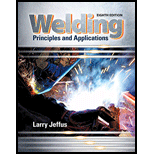# What are two ways math is most commonly used in the welding shop?### Welding: Principles and Applicatio...

8th Edition
Larry Jeffus
Publisher: Cengage Learning
ISBN: 9781305494695### Welding: Principles and Applicatio...

8th Edition
Larry Jeffus
Publisher: Cengage Learning
ISBN: 9781305494695

#### Solutions

Chapter
Section
Chapter 20, Problem 1R
Textbook Problem

## What are two ways math is most commonly used in the welding shop?

Expert Solution
To determine

To Explain: Two ways of math which is used in welding shop.

### Explanation of Solution

Mathematics is important in all the areas for calculating the parameters of the operations. In a welding shop, the operator needs to calculate the angles and position of the torch through mathematics.

There are two main ways in which math is most commonly used in welding shop. They are:

1. Arithmetic operators: addition, subtraction, multiplication and division.
2. Geometrical and trigonometry: Dimensions of the weldments, angle of electrode, point of contact and gap between electrode and work piece.

### Want to see more full solutions like this?

Subscribe now to access step-by-step solutions to millions of textbook problems written by subject matter experts!

Get Solutions

### Want to see more full solutions like this?

Subscribe now to access step-by-step solutions to millions of textbook problems written by subject matter experts!

Get Solutions

Find more solutions based on key concepts
Show solutions
What mode is used to manually enter programs into the machine control?

Precision Machining Technology (MindTap Course List)

Explain the two-step verification process.

Enhanced Discovering Computers 2017 (Shelly Cashman Series) (MindTap Course List)

How do you display text and values in MATLAB?

Engineering Fundamentals: An Introduction to Engineering (MindTap Course List)

What are the two main categories of UPSes?

Network+ Guide to Networks (MindTap Course List)

What is a sequence? Write its syntax.

Database Systems: Design, Implementation, & Management

If your motherboard supports ECC DDR3 memory, can you substitute non-ECC DDR3 memory?

A+ Guide to Hardware (Standalone Book) (MindTap Course List)